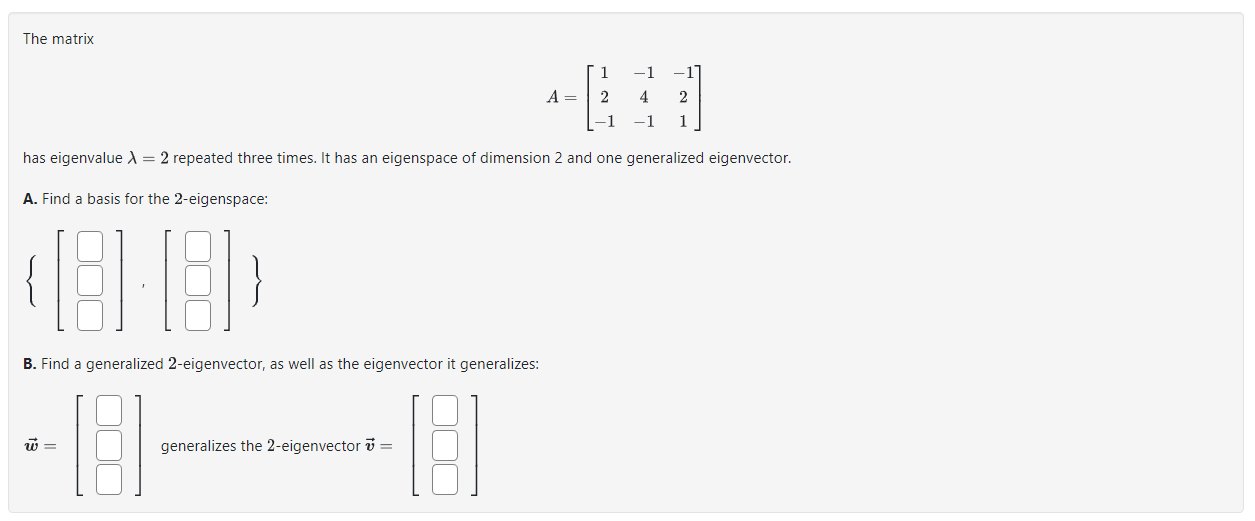Home / Expert Answers / Other Math / a-left-begin-array-ccc-1-1-1-2-4-2-1-1-1-end-array-right-has-ei-pa828

# (Solved): $A=\left[\begin{array}{ccc} 1 & -1 & -1 \\ 2 & 4 & 2 \\ -1 & -1 & 1 \end{array}\right]$ has ei ...$A=\left[\begin{array}{ccc} 1 & -1 & -1 \\ 2 & 4 & 2 \\ -1 & -1 & 1 \end{array}\right]$ has eigenvalue $$\lambda=2$$ repeated three times. It has an eigenspace of dimension 2 and one generalized eigenvector. A. Find a basis for the 2-eigenspace: $\{[-],[-]\}$ B. Find a generalized 2-eigenvector, as well as the eigenvector it generalizes: $\overrightarrow{\boldsymbol{w}}=[=] \text { generalizes the 2-eigenvector } \overrightarrow{\boldsymbol{v}}=[-]$

We have an Answer from Expert